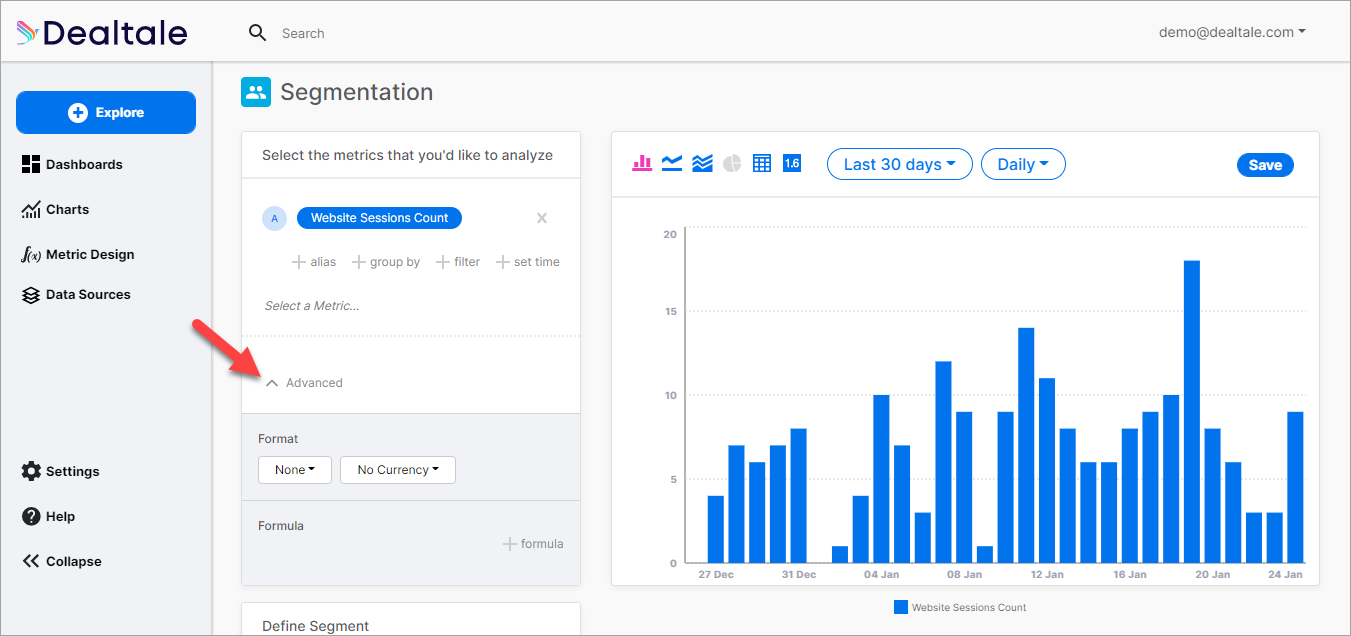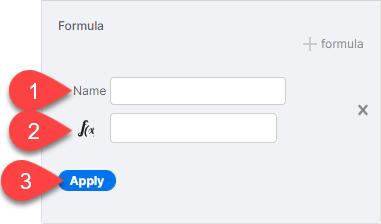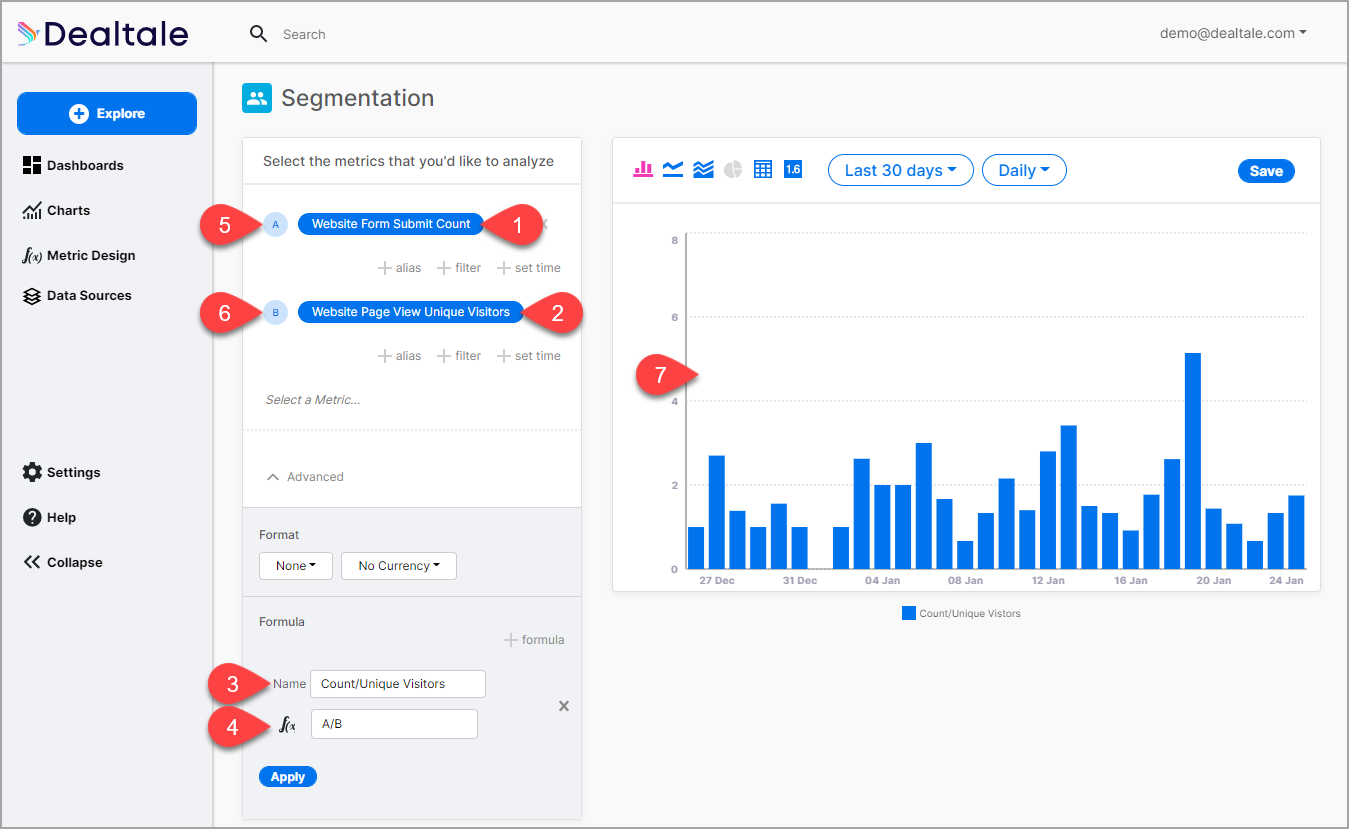# Configuring Advanced Settings

Create custom calculation in the Advanced section of the Segmentation page.# Format

You can format how to display your data on your chart according to number formatting and currency.

# Formula

You can use the Formula feature to calculate different Metrics and get specific results that are reflected on the chart on the right.

Note: You must select two or three metrics to be able to calculate a formula. Refer to the Selecting Another Metric section in Using KPI Analysis for instructions.

1. Name your Formula in the field provided (1).1. Enter a formula in the f(x) field (2).
2. Click Apply (3).In our example shown in the image above, we selected Count in the first Metric (1) and Unique Visitors in the second Metric (2). We named the Formula "Count/Unique Visitors."(3)

We now want to compute the number of submitted website forms compared to the number of unique visitors so we use the formula A divided by B (4):

``````A/B

whereby:

A (5), the Count of Website Form Submit is divided by
B (6), the number of Website Page View Unique Visitors.
``````

After clicking Apply, the result of the formula reflects on the chart on the right (7).